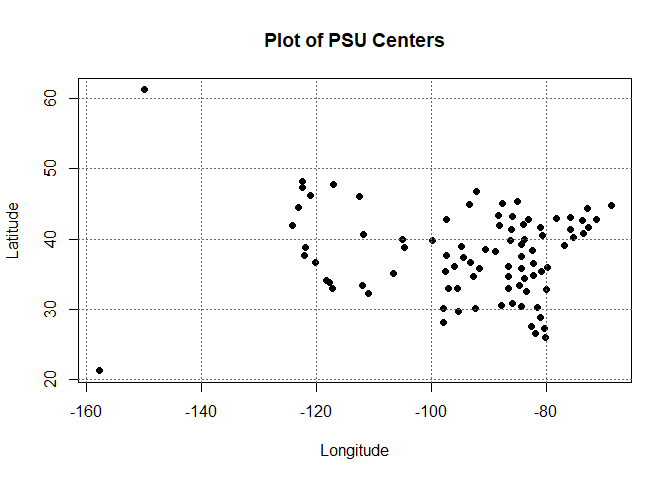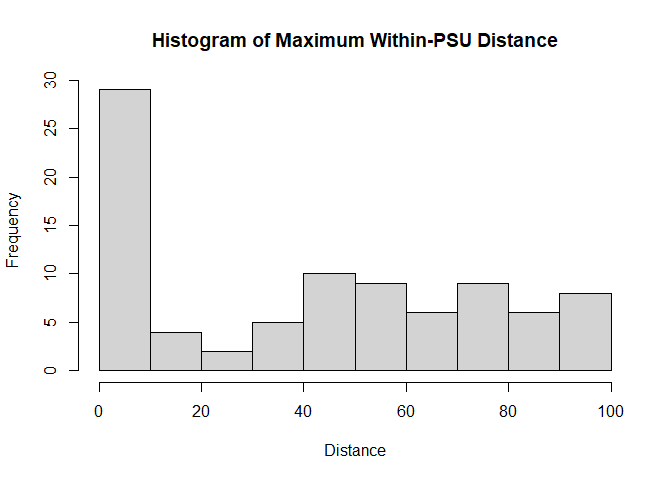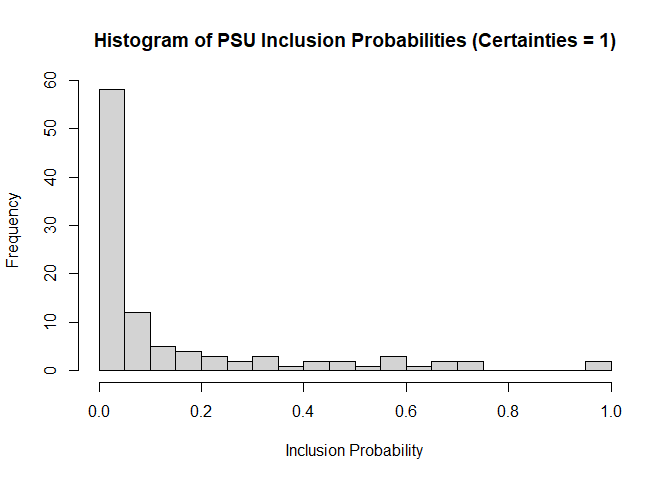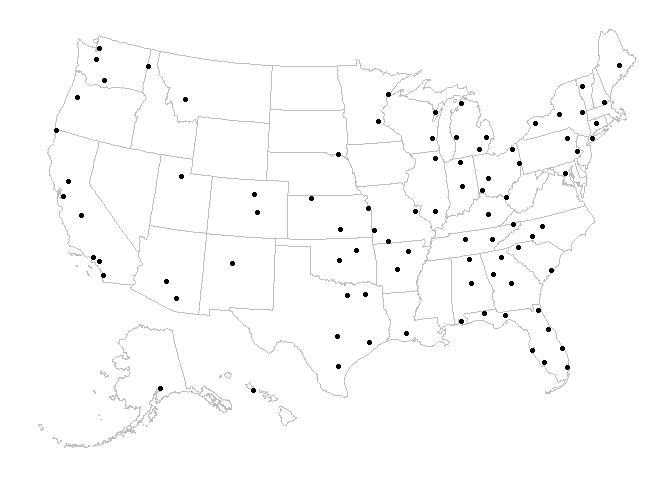# Creating Geography-Based PSUs with as Similarly-Sized MOS as Possible

#### 2023-05-22

In a survey population of geographic sampling units, it may be required that a sampling frame of primary sampling units (PSUs) be developed where geographic sampling units are combined. This is particularly true in two-stage sample designs, where central geographic units may be chosen for a team of survey researchers to visit and secondary stage sampling units (SSUs), e.g. households, schools, or businesses, are the units of interest. In this vignette, we review how to use the GeoDistPSU and GeoDistMOS functions in the PracTools package to create a sampling frame of geography-based PSUs and show an example. We provide some guidelines in defining PSUs, how to display the United States geography-based PSUs with maps, and also show how the results of geography-based sample frame can be integrated with two-stage sample size calculations.

## Guidelines for Creating Geography-Based PSUs

The following are useful guidelines for creating geography-based PSUs.

1. Political Boundaries. There are many situations where it makes sense to use political boundaries, e.g. states or counties, to separate geographic sampling units. GeoDistPSU does not separate sampling units based on political boundaries. If this step is necessary, it may make sense to split the data into politically-based data sets and apply GeoDistPSU on each politically bounded data set accordingly.
2. Maximum Distance. The area of a geography-based PSU should not exceed a maximum distance. This parameter can be used to limit the amount of within-PSU traveling that a data collector must do. Maximum distance is a required setting in GeoDistPSU. The user must select between “miles” and “kms” (kilometers) for the distance metric.
3. Measure of Size (MOS). The MOS can be different quantities depending on the application, e.g., number of housing units or persons for a household survey or total dollar amount of accounts in an auditing sample. Ideally, each geography-based PSU would have a similar measure of size for sample selection purposes. In practice, there are likely to be geographic units whose MOS’s are so large that the units are certainties. However, it is sometimes possible to split certainties into smaller geography-based PSUs. GeoDistMOS splits extremely large PSUs based on a user-defined MOS take-all threshold.

## Data Requirements for Geography-Based PSUs

GeoDistPSU requires that each geographic sampling unit contain a specific longitude and latitude in decimal format. Where the geographic sampling units are areas larger than a specific location, e.g. counties or census enumeration areas, then a specific longitude and latitude must be selected. Typically, the specific longitude and latitude is the centroid for the geographic sampling unit. However, other considerations may include importance of political entities such as capitals or other centers of government, population densities within the geographic sampling area, whether the geographic center of the sampling area is appropriate, etc.

GeoDistMOS requires a Measure of Size variable and a threshold value above which a geography-based PSU is a certainty.

## Process for Creating Geography-Based PSUs

The process for creating geography-based PSUs with similar MOS’s is as follows:

1. Ensure each geographic sampling unit has a longitude and latitude in decimal format. If the longitude and/or latitude are character or missing, the function will not work. It is recommended that the data be plotted or otherwise reviewed prior to creating geography-based PSUs as errors in geocoding can occur. If the data does not have the longitude and latitude coordinates, the R package tidygeocoder can be used to perform geocoding. The vignette for the tidygeocoder package, (Cambon et al. 2021), provides an overview on how to use it. Again, check the results of tidygeocoder to ensure that all geographic sampling units have the correct latitude and longitude before using the GeoDistPSU function.
2. Use GeoDistPSU function. The GeoDistPSU function requires the following: Longitude, Latitude, a switch for either “miles” or “kms” (kilometers), and a maximum within-PSU distance value. GeoDistPSU output two data frames. The data frame PSU.ID provides the geography=based PSU (psuID) and the Input.ID for merging to the original input file if there is one. The other data frame, PSU.Info, contains geography-based PSU-level information. For example, output from this data frame can create a plot of the PSU centroids. A histogram of the maximum within-PSU distance can also provide useful information to the survey practitioner.
3. If the sample design is probability proportional to size (pps), then it may be useful to apply the GeoDistMOS function. The GeoDistMOS function requires the following: Longitude, Latitude, PSU ID, the sample size, the MOS variable, and the threshold value for certainties. The threshold value is a probability in the range (0, 1]; PSUs with a selection probability greater than or equal to the threshold are certainties. It is assumed that the sampling units in a PSU ID already are within the maximum PSU distance. If the PSU ID is taken from GeoDistPSU, then this is guaranteed. It is recommended that a histogram of the final MOS be reviewed.

## Example

The data set Test_Data_US comes from the US Department of Transportation. Test_Data_US already has the longitude and latitude in decimal format. The amount variable will be used for the MOS part of the example. To create geography-based PSUs that have a maximum distance of 100 miles, the code is:


library(PracTools)
data(Test_Data_US)
GeoDistPSU(Test_Data_US$lat, Test_Data_US$long, "miles", 100, Input.ID = Test_Data_US$ID) If the GeoDistPSU function is saved to an object, then a plot of the centroids and a histogram of the maximum distances may be generated. To do that, the code is:  g <- GeoDistPSU(Test_Data_US$lat, Test_Data_US$long, "miles", 100, Input.ID = Test_Data_US$ID)

plot(g$PSU.Info$PSU.Mean.Longitude,
g$PSU.Info$PSU.Mean.Latitude,
pch  = 19,
main = "Plot of PSU Centers",
xlab = "Longitude",
ylab = "Latitude")
grid(col = "grey40")hist(g$PSU.Info$PSU.Max.Dist,
main = "Histogram of Maximum Within-PSU Distance",
xlab = "Distance",
ylab = "Frequency")The plot shows the PSU cluster centroids. As can be seen, a vague outline of the United States is visible, with Alaska in the upper left and Hawaii in the lower left. The section on displaying United States geography-based PSUs shows how to add a political map to this.

The histogram provides a bar chart of the maximum within-PSU distance. As can be seen, no PSU contains a maximum distance of more than 100 miles. In this data set, there are many PSUs with a maximum distance of zero miles. This is because the geography-based sampling unit in the PSU was more than 100 miles from its next nearest geography-based sampling unit; it becomes its own PSU under the distance rules.

While GeoDistPSU creates PSUs with a maximum distance, the function does not address measure of size. For a pps sample design, some PSUs may need to be split to create a more even distribution of MOS across the PSUs. Here, the PSU ID is added to the Test_Data_US from the GeoDistPSU output and then input into GeoDistMOS. Let’s assume the sample size is 15 and the take-all threshold is 0.80. The code is:


Test_Data_US$psuID <- g$PSU.ID$psuID ## Update GeoDistPSUs for a threshold measure of size of 0.80 m <- GeoDistMOS(lat = Test_Data_US$lat, long = Test_Data_US$long, psuID = Test_Data_US$psuID, n = 15, MOS.var = Test_Data_US$Amount, MOS.takeall = 0.80, Input.ID = Test_Data_US$ID)

Note that the sample size of PSUs, n=15, may be a preliminary value used in the computation to identify certainties. The final sample size may be determined after computing relvariance components using the PSUs that are formed with GeoDistPSU or GeoDistMOS as illustrated later in the vignette.

The list object created by GeoDistMOS contains two data frames. The first data frame, PSU.ID.Max.MOS contains the original PSU ID for the geographic sampling units, their new PSU ID based on the maximum measure of size threshold, and the Input ID for merging to the original data file.

The second data frame, PSU.Max.MOS.Info, contains the information on the updated PSU ID given the MOS threshold, the inclusion probability for the updated PSU ID given the sample size used for GeoDistMOS, and the number of SSUs in each PSU. The inclusion probabilities can be shown in a histogram to assess of the distribution of the MOS values. R’s descriptive functions like summary() and var() also give useful statistics. For example, if the variance of the MOS is zero, PSUs all have the same size.

A histogram of the MOS for the updated PSUs can be generated with the following code:


hist(m$PSU.Max.MOS.Info$psuID.prob,
breaks = seq(0, 1, 0.05),
main = "Histogram of PSU Inclusion Probabilities (Certainties = 1)",
xlab = "Inclusion Probability",
ylab = "Frequency")This histogram allows the survey practitioner to see how evenly sized the PSUs are. The constraint of the maximum travel distance being 100 miles within each PSU limits shows how well the “evenly sized” goal can be met. Here, while slightly over two thirds of the MOS are less than 0.10, there is something of a tail that the survey practitioner might want to consider. As an aside, the default for hist() bars is a left-open, right-closed interval. In this histogram, all certainties equal to 1 are in the bar between 0.95 and 1.00. For other approaches to displaying the inclusion probabilities, the reader is referred to ggplot vignettes.

## Displaying Geography-Based PSUs

The output from GeoDistPSU is a list. Mapping in R almost always requires a data frame. The R package usmap can be used to overlay the United States political boundaries on the PSU centroids. The usmap package requires that the longitude and latitude coordinates of the centroids be in a data frame which is then transformed by application of the Albers Equal Area projection. The code is below. (Uncomment the code to run it.)

#library(sp)
#library(usmap)
#library(ggplot2)
## Transform PSUs into usmap projection
#g.map  <- cbind(long = g$PSU.Info$PSU.Mean.Longitude,
#          lat  = g$PSU.Info$PSU.Mean.Latitude)
#g.map  <- as.data.frame(g.map)
#g.proj <- usmap::usmap_transform(g.map,
#             input_names  = c("long", "lat"),
#             output_names = c("Long", "Lat"))
#plot_usmap(color = "gray") +
#        geom_point(data = g.proj, aes(x = Long, y = Lat))US map with PSUs

As can be seen, this code gives the survey practitioner working with United States geographic sampling units to immediately identify where the PSUs are. For additional improvements on the plot, such as labels, the reader is referred to ggplot vignettes.

## Geography-Based PSUs and Two-Stage Sample Size Calculations

PracTools comes with two functions that are helpful with two-stage sample size calculations: BW2stageSRS and BW2stagePPS. BW2stageSRS computes the relvariance components for a two-stage sample where both PSUs and elements within PSUs, or Secondary Sampling Units (SSUs), are selected via simple random sample (SRS). BW2stagePPS computes the relvariance components for a two-stage sample where the PSUs are selected with probabilities proportional to size (PPS) and the SSUs are selected via simple random sample. Both functions require that an entire sampling frame be used as input. The values from these functions can be used to optimize the number of first-stage and second-stage sample units. This section reviews these two functions based on the output from GeoDistPSU, and then again with the output from GeoDistMOS. As the primary sampling unit ID, we use the field PSU, which was appended to Test_Data_US above.

To understand what BW2stageSRS and BW2stagePPS are doing, we can examine the formula for the relvariance of an estimator of total. Suppose that $$\small m$$ is the number of sample PSUs and that the same number of SSUs, $$\small \bar{n}$$, is selected in each sample PSU. The approximate relvariance of an estimator of a total with either SRS at both stages or pps at the first stage and SRS at the second can be written as:

$\begin{equation} \small \frac{V(\hat{t}_{\pi})}{t^2_U} = \frac{B^2}{m} + \frac{W^2}{m\bar{n}} = \frac{\tilde{V}}{m\bar{n}}k[1 + \delta(\bar{n} - 1)]\;. \end{equation}$

where $$\small B^2$$ is the between-PSU sum of squares and $$\small W^2$$ is the within-PSU sum of squares. $$\small \tilde{V}$$ is the unit relvariance of the analysis variable, $$\small \delta = B^2/(B^2 + W^2)$$, and $$\small k = (B^2+W^2)/\tilde{V}$$. The equation above applies when the sampling fractions are negligible at both stages. See Valliant, Dever, and Kreuter (2018), sections 9.2.1 and 9.2.3 for details.

In preparing data for the BW2stageSRS and BW2stagePPS, it is important to consider which variable is used for the relvariance calculations in a two-stage sample. Test_Data_US$Y is used in this example, but different analysis variables will have different components. For BW2stagePPS, the inclusion probabilities are also necessary. GeoDistPSU creates PSUs so that the maximum travel distance within a PSU is constrained without considering any measure of size associated with the input units. After adding the PSU from GeoDistPSU to the data frame (code shown above), the code for BW2stageSRS is:  BW2stageSRS(X = Test_Data_US$Y, psuID = Test_Data_US$psuID, lonely.SSU = "zero") #> B2 W2 unit relvar B2+W2 k delta #> 2.0201042 3.3777276 1.4277924 5.3978318 3.7805439 0.3742436 To perform the calculations using BW2stagePPS, it is necessary to have a vector of 1-draw inclusion probabilities for the PSUs, i.e., the selection probability of each PSU in a sample of size 1. For the MOS variable, we use the field, Amount, in Test_Data_US. Thus, this example illustrates the effects of selecting the PSU sample with probabilities proportional to Amount and computing relvariance components for Y. The code for BW2stagePPS, using the PSUs created by GeoDistPSU, is:  pp <- tapply(Test_Data_US$Amount, Test_Data_US$psuID, sum)/sum(Test_Data_US$Amount)
BW2stagePPS(X = Test_Data_US$Y, pp = pp, psuID = Test_Data_US$psuID, lonely.SSU = "zero")
#>          B2          W2 unit relvar       B2+W2           k       delta
#>   0.5450555   0.9638436   1.4277924   1.5088991   1.0568057   0.3612273

Delta is a within-PSU measure of homogeneity and is defined as the between sum of squares divided by the sum of the between sum of squares and the within sum of squares; as noted above, $$\small \delta = B^2/(B^2 + W^2)$$. The closer the MOS is to be being proportional to the variable of interest being collected in the sample, the smaller $$\small B^2$$ will be in a PPS sample. The extreme case of $$\small \delta$$ near zero implies only one PSU needs to be sampled as each PSU is statistically identical. In this example, the delta values are similar for both SRS and PPS, although that will often not be the case.

The term $$\small k$$ is the ratio of $$\small B^2+W^2$$ to the unit relvariance of Y. If $$\small k$$ is close to 1, then there is not much variation in the size of the secondary sampling units within each PSU. In fact, the variance formula for a single-stage cluster sample assumes $$\small k = 1$$. However, if $$\small k$$ is not close to 1, then the survey estimates in a two-stage SRS sample may not be very precise for some variables where the PSUs vary in size. As can be seen from the equation for the relvariance of an estimated total above, the lower $$\small k$$ for BW2stagePPS suggests PPS sampling may be preferred even though the $$\small \delta$$’s in SRS and PPS are about the same.

The output from GeoDistMOS, which evens out the PSU MOS sizes to the extent feasible, may provide additional information. This is because creating clusters of equal sizes should be performed, if possible. Large variations in PSU sizes is likely to result in large variations in the PSU estimated totals. Large variations in the estimated PSU totals in turn lead to a high between sum of squares relative to the within sum of squares. This in turn leads to a high measure of PSU homogeneity and a less efficient two-stage sample.

Combining the output from the PSU.ID.Max.MOS data frame with the original data frame can be done by merging on the Input.ID field. GeoDistPSU and GeoDistMOS output the ID field as a character. In this example, the original ID field is numeric. To combine:


library(dplyr)
Test_Data_US <- Test_Data_US %>% mutate(ID = as.character(ID))
Test_Data_US <- inner_join(Test_Data_US, m$PSU.ID.Max.MOS, by=c("ID" = "Input.ID")) With the geographic data frame updated for a more equal measure of size by GeoDistMOS, re-running the above returns:  BW2stageSRS(X = Test_Data_US$Y, psuID = Test_Data_US$psuID.new, lonely.SSU = "zero") #> B2 W2 unit relvar B2+W2 k delta #> 1.4712848 1.8843484 1.4277924 3.3556331 2.3502249 0.4384522 We can also compute components with the PSUs created by GeoDistMOS but assuming that PSUs are selected via PPS. We first note that two of the PSUs formed by GeoDistMOS will be certainties since their values of m$PSU.Max.MOS.Info$psuID.prob is approximately 1. These PSUs can be identified with this code:  certs <- (1:nrow(m$PSU.Max.MOS.Info))[m$PSU.Max.MOS.Info$psuID.prob > 0.8]
certID <- m$PSU.Max.MOS.Info[certs, "psuID.new"] certID #>  "1.1" "2.1" The certainties have psuID.new’s of 1.1 and 2.1. Because a PSU selected with probability 1 will not contribute to the $$\small B^2$$ component of variance, we exclude them before calling BW2stagePPS.  pp <- tapply(Test_Data_US$Amount, Test_Data_US$psuID.new, sum)/sum(Test_Data_US$Amount)
sub.Test_Data_US <- Test_Data_US[!(Test_Data_US$psuID.new %in% certID),] sub.pp <- pp[-certs] BW2stagePPS(X = sub.Test_Data_US$Y, pp = sub.pp, psuID = sub.Test_Data_US\$psuID.new,
lonely.SSU = "zero")
#>          B2          W2 unit relvar       B2+W2           k       delta
#>   0.6537961   0.9631122   1.1043456   1.6169083   1.4641326   0.4043495

As can be seen, making the clusters more evenly sized increases the delta slightly. For the data here, it does seem that a first-stage PPS sample is the better approach based on its k-value being close to 1, and that evening the size of the MOS does increase heterogeneity in the sampling frame. Finally, to optimize the sample sizes at each stage in a two-stage sample design, the reader is referred to PracTools::clusOpt2.